# Heat Pipe Calculator Use Instructions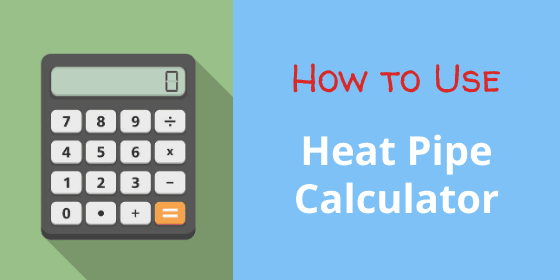Our online heat pipe calculator provides the following heat pipe performance data: heat pipe thermal conductivity by diameter, heat pipe carrying capacity (Qmax) by diameter & orientation, and delta-T from one end of the heat pipe to the other. From this last calculation, it’s simple to arrive at the thermal resistance of the heat pipe. All calculations are for a copper heat pipe using sintered wick material and water as the working fluid.

Here’s a link to the actual heat pipe calculator.

## Heat Pipe | Heat Sink Input Section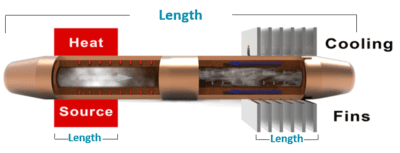Heat PIpe Calculator Inputs

Heat Pipe Length – the full length of the heat pipe if the evaporator is at one end.

Evaporator Length – the evaporator length is the length of the actual heat source.

Condenser Length – the distance between the points where the heat pipe enters and exits the condenser.

Heat Pipe Type – Select the heat pipe wick material ‘Standard’ or ‘Performance’ sintered wick material. Standard wicks will allow you to flatten the heat pipe more before affecting the maximum power (Qmax). Note that we can alter the heat pipe wick porosity and thickness to closely match the requirements of your application, although it is not shown here.

Operating Temperature – This is the temperature of the vapor inside of the heat pipe. It is difficult to know this number and the accuracy of this input is not critical. However, use the average of the Tmax ambient and the Tmax case temperature. Sample calculation:  50 oC Max Ambient, 95 oC  Max Case  = (50 + 95)/2 = 72.5 oC Operating Temperature.

## Heat Pipe Results Section

### Heat Pipe Effective Thermal Conductivity

The first table calculates heat pipe effective thermal conductivity from 3-10mm diameter. We probably should have put this last as it’s used once you’ve selected the correct diameter. Nonetheless, this figure is used as input into Excel and/orCFD modeling software such as FloTHERM. Read more about heat pipe thermal conductivity.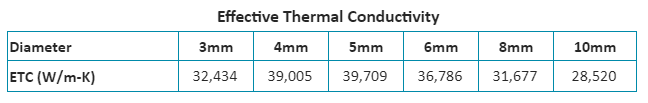Heat Pipe Thermal Conductivity

### Heat Pipe Carrying Capacity (Qmax)

Next, there is a Power vs Angle of Operation graph (shown) and corresponding table (not shown). Essentially this is giving you the maximum heat pipe carrying capacity (Qmax) of a particular diameter heat pipe at various angles.  At “+90” degrees the condenser is directly above the evaporator, making it very easy for the condensed vapor (water) to return to the evaporator, hence the high Qmax.

There are a couple of heat pipe design guidelines that will come in handy at this point.

• First, heat pipe Qmax is additive, provided each heat pipe(s) is over the heat source. In our example, this means that one 8mm heat pipe has a Qmax of 62 oC in horizonal operation, while two 8mm pipes have a Qmax of 124 oC .
• Second, build in a safety factor to avoid running the pipe at maximum capacity. Derating the heat pipe Qmax by 20-25% is a good industry standard – in this case a single pipe would be rated at just under 50 watts. Occasional, short power spikes above this are OK so long as they are still below the rated Qmax. Click to view our comprehensive Heat Pipe Design Guide.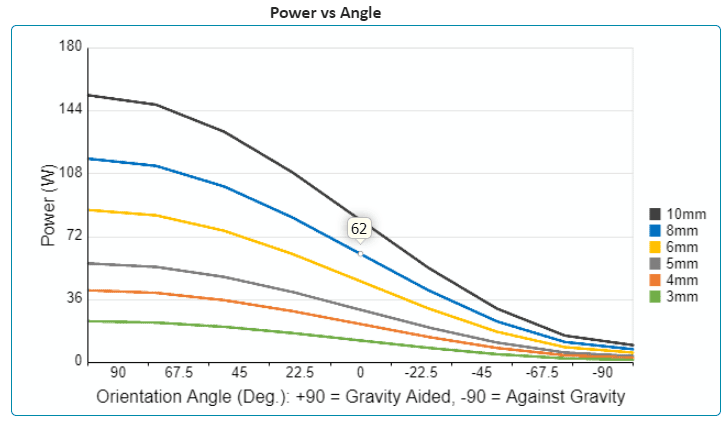Heat Pipe Power Carrying Capacity (Qmax)

### Heat Pipe Thermal Resistance Calculation

The Power vs Delta-T graph and table (shown below) needs to be used in conjunction with the above graph. Let’s say we’ve selected the 8mm heat pipe we talked about earlier: in the horizontal position, it will safely carry just under 50 watts (after being derated).  If we put 40 watts into one end, the other end will have a delta-T of 4.3 oC (lower is better).  Let’s assume we wanted to use two 8mm heat pipes. In that case we could safely double our heat input to 80 watts. However, then using the chart we would still use the a 40 watt input figure because each heat pipe would carry 40 watts and the delta-T of each heat pipe would be the same 4.3 oC . To calculate the thermal resistance of the 8mm heat pipe, simply divide the detla-T by the input power. In this case it would be 4.3/40 = 0.11 oC  per watt.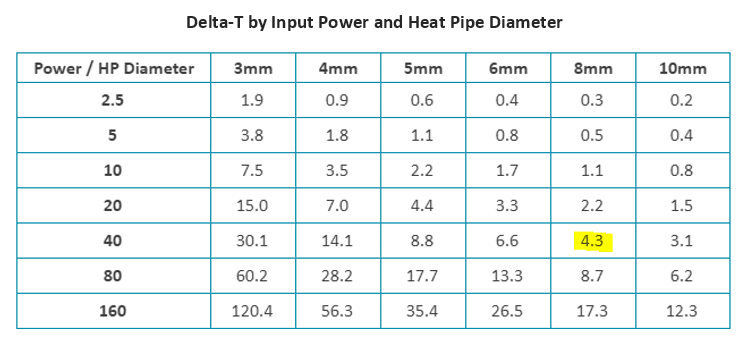Chart Used in Calculating Heat Pipe Thermal Resistance

### Flat Heat Pipe

The last bit of information given isn’t a calculation, it’s simply some guidance on how much you can flatten heat pipes of different diameters before the Qmax will be negatively affected. The reason the performance heat pipes suffer degradation before standard pipes has to do with the thicker wick structure of the former.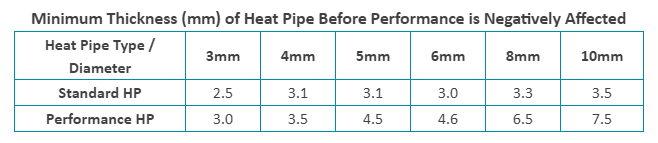We hope you find this online heat pipe calculator useful. Visit our Calculators Page to see the heat sink size and heat sink performance. Links to usage instructions can be found in the calculator or though our Heat Sink Blog Page

Celsia is a custom heat sink manufacturer using vapor chamber and heat pipe technology. We specialize in: heat sink design, heat sink proto, heat sink manufacturing, heat pipes, vapor chambers, custom heat pipe wick materials.